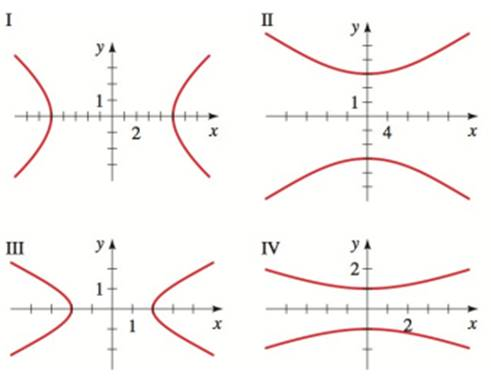Chapter 12.3, Problem 8E### Algebra and Trigonometry (MindTap ...

4th Edition
James Stewart + 2 others
ISBN: 9781305071742

#### Solutions

Chapter
Section### Algebra and Trigonometry (MindTap ...

4th Edition
James Stewart + 2 others
ISBN: 9781305071742
Textbook Problem

# SKILLSGraphs of Hyperbolas Match the equation with the graphs labeled I − I V . Give reasons for your answers. 9 x 2 − 25 y 2 = 225To determine

The graph for the given hyperbola.

Explanation

Given:

Equation of hyperbola,

9x225y2=225

Approach:

The form of hyperbola equation with horizontal transverse is given by,

x2a2y2b2=1 or x2a2+y2b2=1

Calculation:

The given equation can be converted into standard hyperbola equation as follows:

9x225y2=2259x222525y2

### Still sussing out bartleby?

Check out a sample textbook solution.

See a sample solution

#### The Solution to Your Study Problems

Bartleby provides explanations to thousands of textbook problems written by our experts, many with advanced degrees!

Get Started